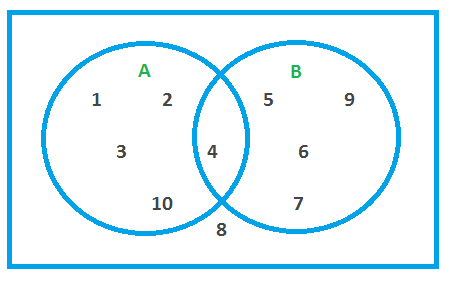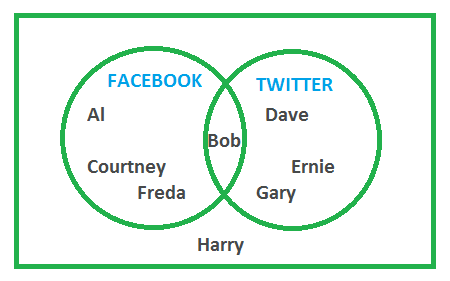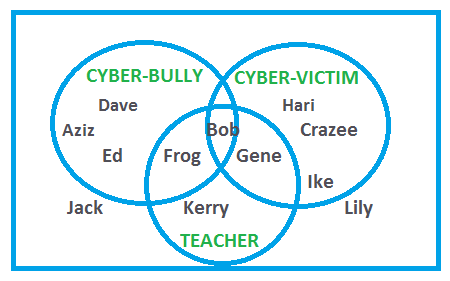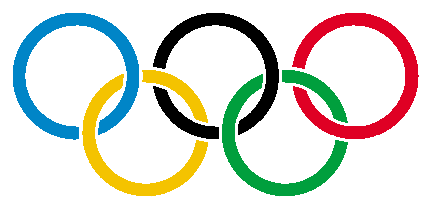# Venn Diagrams

Venn diagrams, also called set diagrams, are helpful in calculating probabilities.

Symbols used include:

• ∪ = union of sets
• ∩ = intersection of sets
• A = complement of set A (the elements that are not in set A)
• ∅ = null or empty set

## Example OneThe Venn diagram shows two sets called set A and set B:

Elements of set A = { 1, 2, 3, 4, 10 }
Elements of set B = { 4, 5, 6, 7, 9 }

A ∪ B is the union of sets A and B.
A ∪ B = { 1, 2, 3, 4, 10, 5, 6, 7, 9 }

A ∩ B is the intersection (overlap) of sets A and B.
A ∩ B = { 4 }

A is the complement of set A, that is, the elements that are not in set A.
A = { 5, 6, 7, 9, 8 }

B is the complement of B, that is, the elements that are not in set B.
B = { 1, 2, 3, 4, 10, 8 }

(A ∪ B) is the complement of A ∪ B, that is, the elements that are not in A ∪ B.
(A ∪ B) = { 8 }

(A ∩ B) is the complement of A ∩ B, that is, the elements that are not in A ∩ B.
(A ∩ B) = { 1, 2, 3, 10, 5, 6, 7, 9, 8 }

Note that the elements can be listed in any order.

## Example Two - Social NetworkingThe Venn diagram shows the names of students who use social networking sites of Facebook (set F) and Twitter (set T):

Elements of set F = { Al, Bob, Courtney, Freda }
Elements of set T = { Bob, Dave, Ernie, Gary }

Q1. Which students are in F ∪ T (the union of sets F and T)?
Q2. Which students are in A ∩ B (the intersection of sets F and T)?
Q3. Who is in (F ∪ T) and does not use Facebook or Twitter?

Q4. What is the probability that a student uses only Facebook?
Q5. What is the probability that a student does not use any social network?

A1. F ∪ T = { Al, Bob, Courtney, Freda, Dave, Ernie, Gary }

A2. F ∩ T = { Bob }

A3. (F ∪ T) = { Harry }

A4. Probability of using Facebook only = 38

A5. Probability of not using social network sites = 18

## Example Three - Cyber-BullyingThe Venn diagram shows the names of 12 students in a class at school. Following incidents of cyber-bullying, the teacher drew this Venn diagram of students who are involved in cyber-bullying as Cyber-bullies (set B) and/or Cyber-victims (set V) and the bullying or victimization has to the teacher (set T).

Q1. Which students are bullies?
Q2. Which students are victims?
Q3. Who is both bully and victim?
Q4. Which bullies have been reported to the teacher?
Q5. Which victims have been reported to the teacher?
Q6. Which students who are both bullies and victims have not been reported to the teacher?
Q7. What is Kerry's role in these incidents?
Q8. Which students have no involvement at all?
Q9. What is the probability that a student in this class is a bully?
Q10. What is the probability that a student in this class is both a bully and a victim?
Q11. What is the probability that a student in this class has not been involved in the cyber-bullying incidents in any way?

A1. B = { Aziz, Bob, Dave, Ed, Frog }

A2. V = { Bob, Crazee, Gene, Ike }

A3. B ∩ V = { Bob }

A4. B ∩ T = { Bob, Frog }

A5. V ∩ T = { Bob, Gene }

A6. None. B ∩ V ∩ T = ∅

A7. Kerry is neither a bully nor a victim. Perhaps Kerry is a sensible by-stander who reported the bullying of other students to the teacher.

A8. (B ∪ V ∪ T) = { Jack, Lily }

A9. Probability of being a bully = 512

A10. Probability of being a bully and a victim = 112

A11. Probability of not being involved = 212 = 16

## Question - Party Food

Draw a Venn diagram for the following data.

• 10 people at a party are Al, Bert, Cuc, David, Emon, Freda, Giuseppe, Henri, Ines and Jock.
• Al, Bert and Jock like hamburgers.
• Cuc, David, Emon, Giuseppe, Ines and Jock like pizza.
• Cuc, Ines and Jock like noodles.
• Freda and Henri are vegetarians who do not eat any of the party food.

## Maths Fun - Olympic RingsWrite data about sports for a Venn Diagram in the shape of the five Olympic Rings.